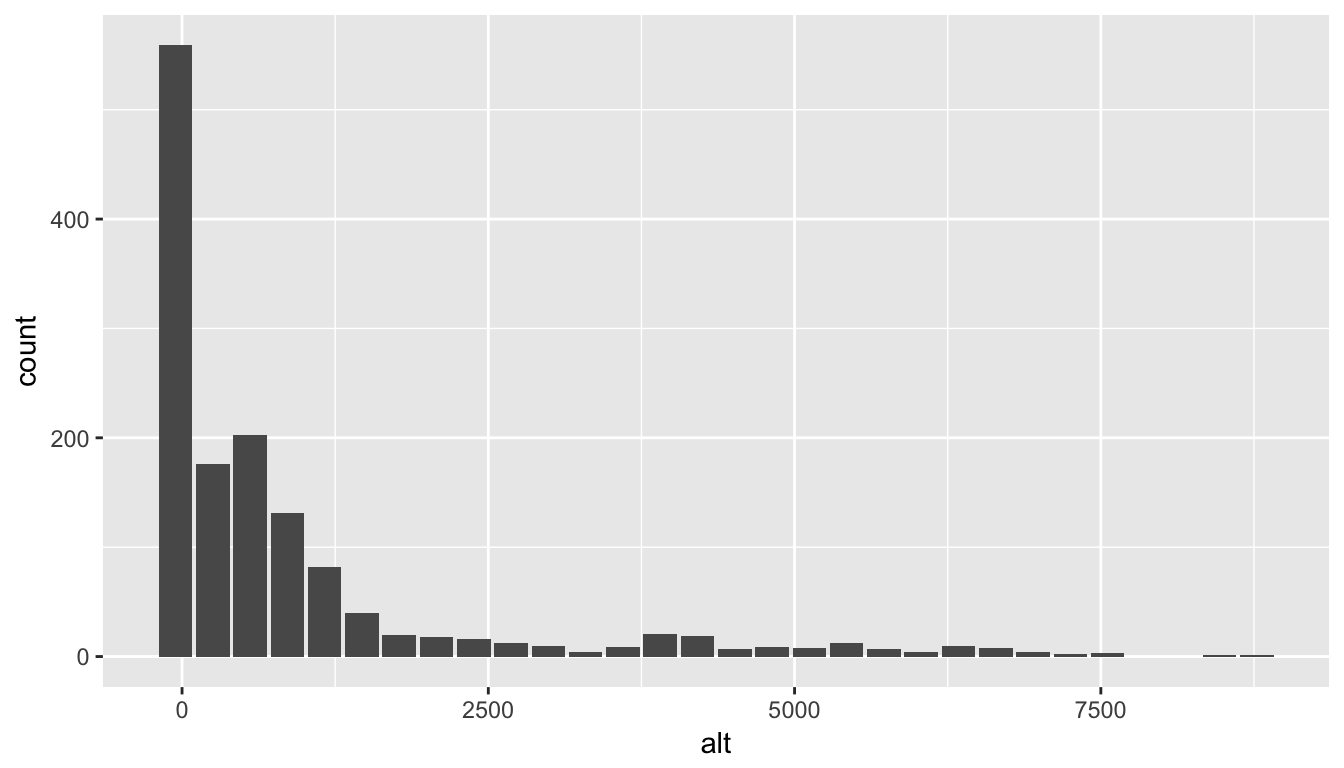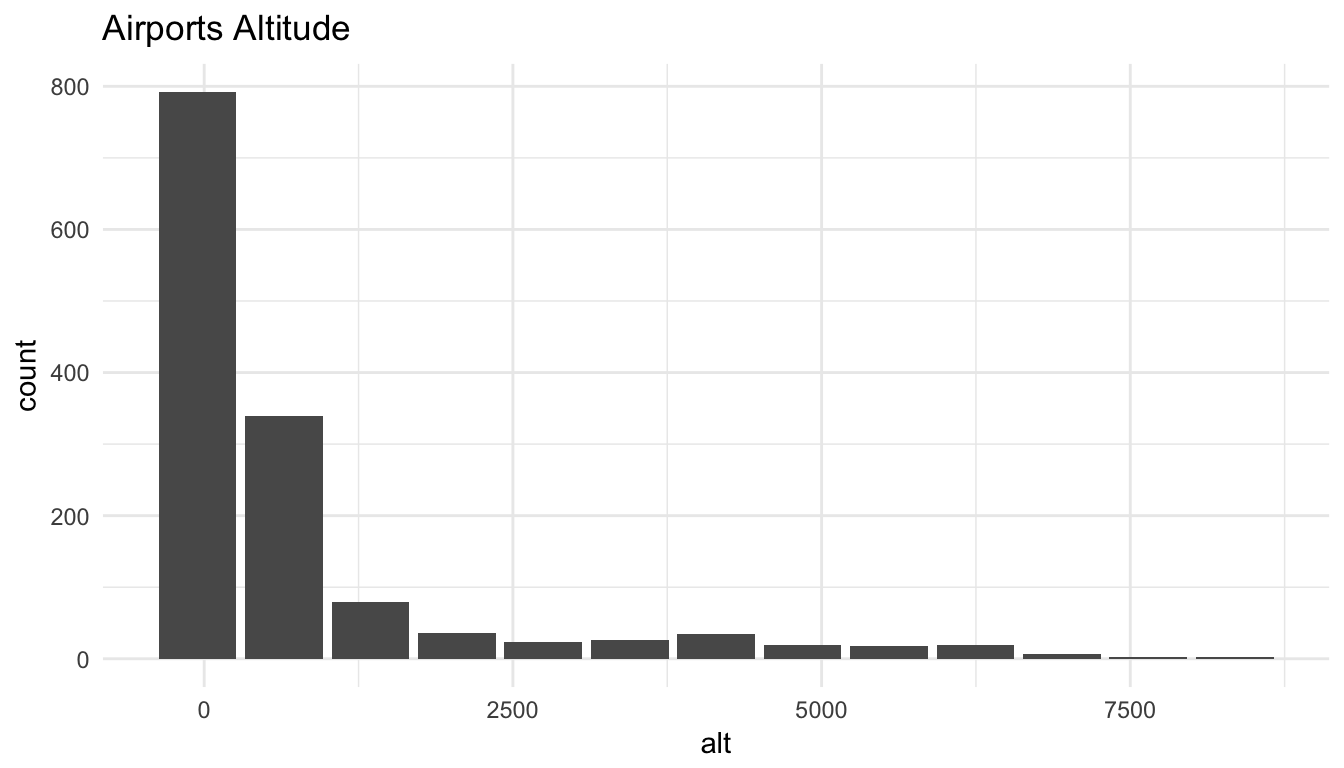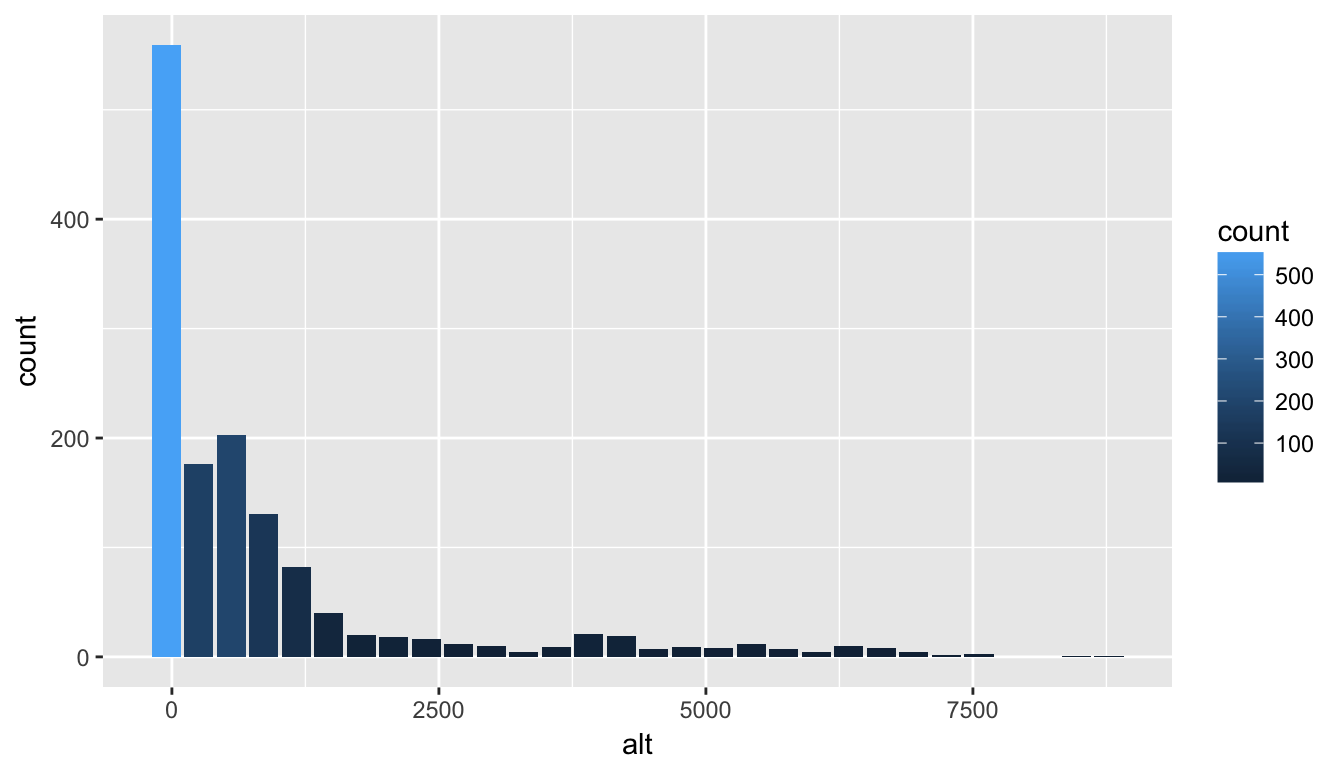# Visualizations with R and Databases

by Edgar Ruiz

## The Challenge

Visualizations are one of R’s strengths. There are many functions and packages that create complex plots, often with one simple command. These plotting functions do two things: first, they take the raw data and run the calculations needed for a given visualization, and second, they draw the plot. If the source of the data resides within a database, the usual approach is to import all of the data and then create the plot. This is a problem, especially if the data is large.

A strategy to address this problem is found in the new Database with RStudio website. The Creating Visualizations page outlines a solution that introduces the “Transform in Database, plot in R” concept, and demonstrates its practical implementation. The article focused on knowledge sharing, rather than on providing a tool.

## Introducing dbplot

The new dbplot package is meant to collect multiple functions for in-database visualization code. It implements the principles laid out in the Creating Visualizations page, and it provides three types of functions:

• Helper functions that return a ggplot2 visualization

• Helper functions that return the results of the plot’s calculations

• The db_bin() function introduced in the Creating Visualizations page

The package provides calculations or “base” ggplot2 visualizations for the following:

• Bar plot

• Line plot

• Histogram

• Raster

## Installation

Install dbplot from GitHub using the devtools package

devtools::install_github("edgararuiz/dbplot")

## Example

This example will use a Microsoft SQL Server database connection to provide a quick glance of how the package works. For more examples, please visit the package’s GitHub repository.

### dbplot functions

The dbplot_histogram() function creates a 30-bin histogram by default. Because it uses dplyr commands to perform the bin calculations, the function will work with any database that has dplyr support, including sparklyr. The only caveat is that the database must support basic functions like max() and min(), which some database types do not support.

library(dbplyr)

tbl(con, "airports") %>%
dbplot_histogram(alt)This example shows how the resulting plot object can be further refined after the dbplot_histogram() function returns a plot:

tbl(con, "airports") %>%
dbplot_histogram(alt, binwidth = 700) +
labs(title = "Airports Altitude") +
theme_minimal()### db_compute functions

If more control over the plot is needed, then the db_compute_bins() function returns a data frame with the lowest value of each bin and the record count per bin:

tbl(con, "airports") %>%
db_compute_bins(alt)

## # A tibble: 28 x 2
##       alt count
##     <dbl> <int>
##  1  -54.0   559
##  2  250.4   176
##  3  554.8   203
##  4  859.2   131
##  5 1163.6    82
##  6 1468.0    40
##  7 1772.4    20
##  8 2076.8    18
##  9 2381.2    16
## 10 2685.6    12
## # ... with 18 more rows

The results of the compute command can then be piped into a plot:

tbl(con, "airports") %>%
db_compute_bins(alt) %>%
ggplot() +
geom_col(aes(alt, count, fill = count))### db_bin()

The dbplot package includes the db_bin() function, first introduced in the Creating Visualizations page. For more information, please read the Histogram section.

db_bin(any_field)

## (((max(any_field) - min(any_field))/(30)) * ifelse((as.integer(floor(((any_field) -
##     min(any_field))/((max(any_field) - min(any_field))/(30))))) ==
##     (30), (as.integer(floor(((any_field) - min(any_field))/((max(any_field) -
##     min(any_field))/(30))))) - 1, (as.integer(floor(((any_field) -
##     min(any_field))/((max(any_field) - min(any_field))/(30))))))) +
##     min(any_field)

## Next steps

More plots will be possible as dplyr-to-SQL translations are fine-tuned and enhanced. The dbplot package will be the place where new calculations and plots will be implemented.

Share Comments · · · · · ·